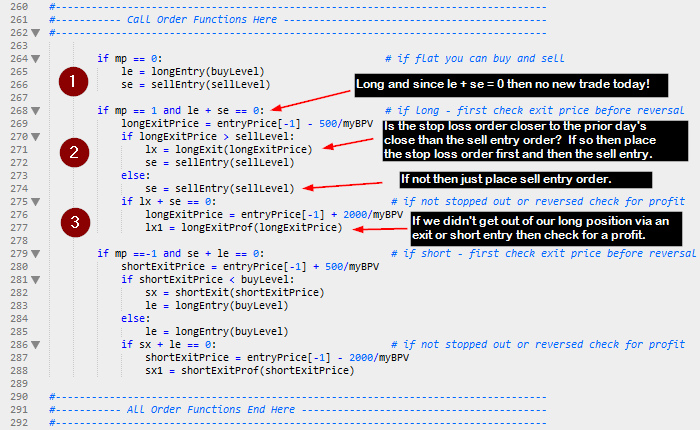# Trade Entry/Exit Functions in PSB

Since Python doesn’t  allow for a GOTO program flow structure I changed the inline trade entry/exit logic into function calls.  This allows for the call of these functions to be non-sequential.  The original PSB order placement was sequential and would examine the entry/exit logic in TOP-DOWN fashion.  Meaning that if you put the Long Entry Logic first, the program would evaluate that logic first on every bar of data.  This was fine for the majority of trading systems out there.  However, systems that could enter multiple signals on the same bar require the orders to be placed in order of whichever was closest to the current market price.  Let’s say you have a trading algorithm that issues a stop to exit at a loss and a stop to reverse your current position.  If you examine the reversal logic prior to the stop loss and the stop loss is actually closer, then you will execute the wrong signal first.   By encapsulating the entry/exit logic into functions you can use decision constructs to flow through the correct logic in the correct order.  Here are the trade signals programmed as function calls:As you can see there are six modules – Long Entry, Long Loss, Long Profit, Short Entry, Short Loss, and Short Profit (note non-necessary code was collapsed).  I used the Sublime text editor to collapse the unnecessary lines of code.  You can download a trail version from their website.  I am no longer using the IDLE as my go to IDE – I have fallen deeply in love with PyScripter.  I will delve into this subject later.

Now that you have all your trade signals programmed as functions you can utilize if-thens to determine what order they are called.  Here is the code that calls these functionsIf you are flat and you can buy or sell on the same day then you call both functions.  This back tester will allow you to buy and sell on the same day – the only problem is it doesn’t know which occurred first: the buy or the sell.  This can cause a problem because you need to know the correct position by the end of the day.  Since we can peak into the future (be very, very careful) we can look at the close of the day and compare it with the long and short entry prices.  Here is some code that might make your entries more accurate on those occasions where both orders could be filled.

``````if myHigh[D0] >= buyLevel and myLow[D0] <= sellLevel:
closeToSellDiff = sellLevel - myClose[D0]
se = sellEntry(sellLevel)
else:
se = sellEntry(sellLevel)
``````
Increasing Trade Entry/Exit Accuracy - Maybe?

This code will execute the long entry logic after the short entry logic whenever the close is closer to the long entry price than the short entry price.  If both orders are filled on the same bar and the close happens to fall closer to the long entry price, then the software assumes the short entry was entered earlier in the day and the long entry later in the day making the position at end of day long.  Is this 100% accurate?  No but it logically stands to reason that a close near the high would indicate they high was made last.  Without intraday data we simply do not know what happened first.

# Using Jupyter Notebook and Plot.ly To Create Candle Stick Chart

In today’s post I show how you can plot a very nice looking Candlestick chart inside a Jupyter (IPython) notebook. This chore is
made much easier by using  Plotly. So first thing you sholud do is sign up for a free account at Plotly and then download Jupyter Interactive Python notebooks.  I did this in an interactive notebook for demonstration purposes only.  After installing Plotly I was able to import the libraries into my notebook and then call the various functions to graph the data.  I imported numpy, but it wasn’t necessary.  I simply copied some data (CL.CSV) to the subdirectory that held my notebooks and then used the CSV Reader to pull the data into the various lists that the Plotly functions required.  All of the plotting is done in a browser and its interactive.  After creating the PSB I wanted to provide a tool for plotting the data that was being tested.  Jupyter and Plotly are free for non-commercial users.

``````import numpy as np
import datetime
import csv
import plotly.plotly as py
from plotly.tools import FigureFactory as FF
from plotly.graph_objs import *

d = list()
dt = list()
o = list()
h = list()
l = list()
c = list()
v = list()
oi = list()
cnt = 0

with open("CL.CSV") as f:
for row in f_csv:
numCols = len(row)
cnt += 1
d.append(int(row))
dt.append(datetime.datetime.strptime(row,'%Y%m%d'))
o.append(float(row))
h.append(float(row))
l.append(float(row))
c.append(float(row))
v.append(float(row))
oi.append(float(row))

xDate = list()
yVal = list()
indicCnt = 0
for i in range(len(c)-40,len(c)):
xDate.append(dt[i])
sum = 0.0
for j in range(i-9,i):
sum += c[j]
yVal.append(sum/10)

fig = FF.create_candlestick(o, h,l, c, dt)